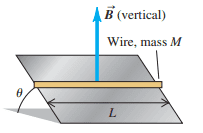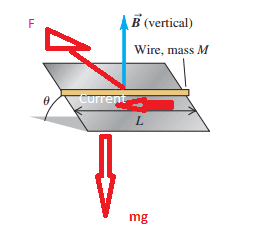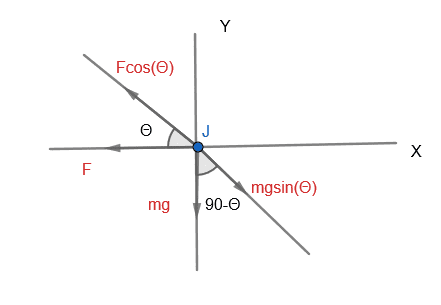# Magnitude and direction of current

• Physicslearner500039

#### Physicslearner500039

Homework Statement
A straight piece of conducting wire with mass and length is placed on a friction less incline tilted at an angle Θ from the horizontal . There is a uniform, vertical magnetic field at all points. To keep the wire from sliding down the incline, a voltage source is attached to the ends of the wire. When just the right amount of current flows through the wire, the wire remains at rest. Determine the magnitude and direction of the current in the wire that will cause the wire to remain at rest.
Relevant Equations
F=ILBsin(Θ) (N); F=mg (N)I am struggling with the angles. Since the conducting wire is moving down for it to be stand still, the force should be opposing it, hence the current should be from Right to Left.I am confident of the Force direction and its value is if ## I ## is the current
##F = ILB (N)## since L and B are perpendicular.
The gravitational force is ##mg (N)## which is acting vertically down. From the previous posts i assume if gravitational force is acting vertically down it does not have the other components. I am confused if the gravitational force has a component along the bar in the downward direction? Please advise.

I am confused if the gravitational force has a component along the bar in the downward direction?
It has. Sketch and end view to see it: the normal force from the incline is, well, normal to the incline, and compensates part of mg and part of F. The other part of the normal force has to be compensated by the other part of F.

•Physicslearner500039
I am confused if the gravitational force has a component along the bar in the downward direction?
What is making the wire slide down the incline?

•Physicslearner500039
Yes now i understand##IBL\cos\theta = mg\sin\theta##
##I = \frac {mg\tan\theta} {BL}## Amps
The ##mg ## component along the X-axis is 0. I got confused with that. Thank You.

•BvU